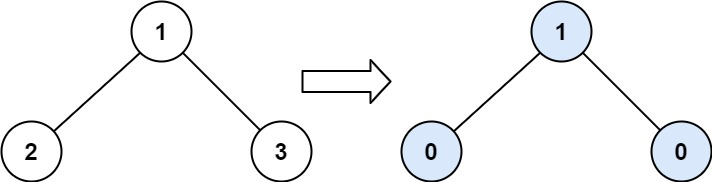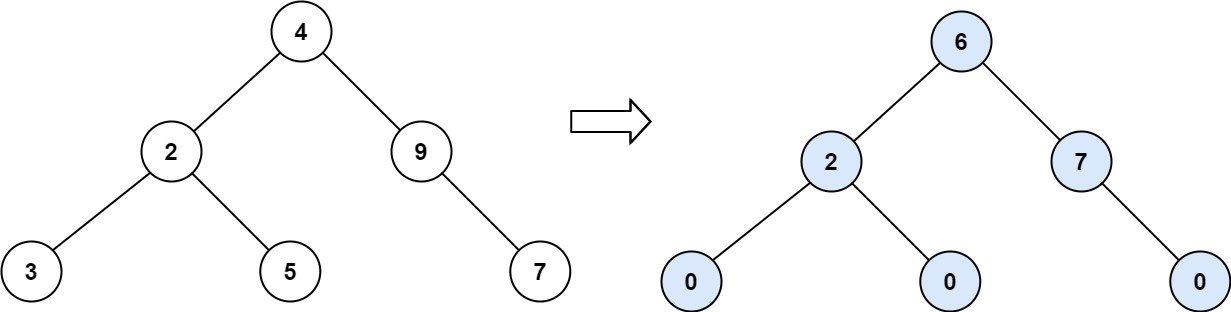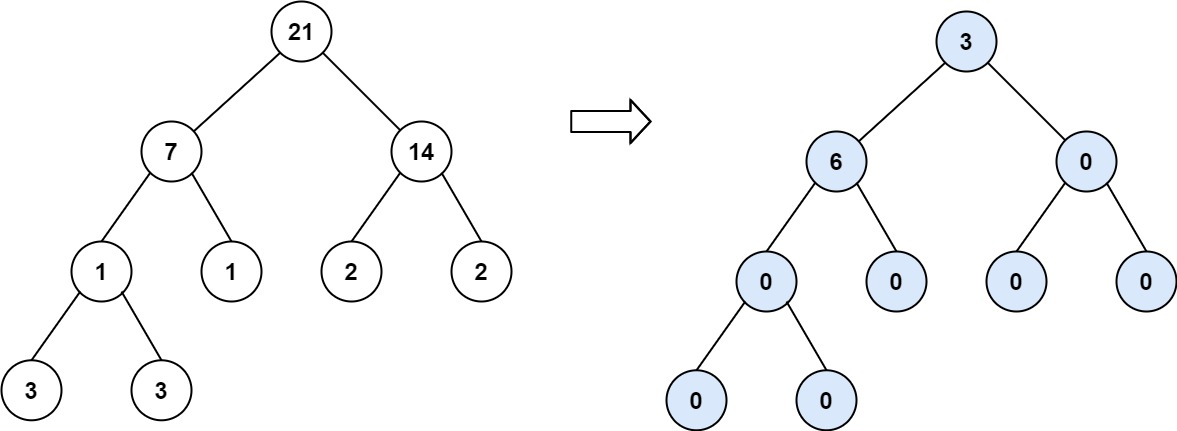## 563. Binary Tree Tilt_soO_007的博客-程序员秘密

Given the `root` of a binary tree, return the sum of every tree node's tilt.

The tilt of a tree node is the absolute difference between the sum of all left subtree node values and all right subtree node values. If a node does not have a left child, then the sum of the left subtree node values is treated as `0`. The rule is similar if there the node does not have a right child.

Example 1:```Input: root = [1,2,3]
Output: 1
Explanation:
Tilt of node 2 : |0-0| = 0 (no children)
Tilt of node 3 : |0-0| = 0 (no children)
Tile of node 1 : |2-3| = 1 (left subtree is just left child, so sum is 2; right subtree is just right child, so sum is 3)
Sum of every tilt : 0 + 0 + 1 = 1
```

Example 2:```Input: root = [4,2,9,3,5,null,7]
Output: 15
Explanation:
Tilt of node 3 : |0-0| = 0 (no children)
Tilt of node 5 : |0-0| = 0 (no children)
Tilt of node 7 : |0-0| = 0 (no children)
Tilt of node 2 : |3-5| = 2 (left subtree is just left child, so sum is 3; right subtree is just right child, so sum is 5)
Tilt of node 9 : |0-7| = 7 (no left child, so sum is 0; right subtree is just right child, so sum is 7)
Tilt of node 4 : |(3+5+2)-(9+7)| = |10-16| = 6 (left subtree values are 3, 5, and 2, which sums to 10; right subtree values are 9 and 7, which sums to 16)
Sum of every tilt : 0 + 0 + 0 + 2 + 7 + 6 = 15
```

Example 3:```Input: root = [21,7,14,1,1,2,2,3,3]
Output: 9
```

Constraints:

• The number of nodes in the tree is in the range `[0, 104]`.
• `-1000 <= Node.val <= 1000`

/**
* Definition for a binary tree node.
* struct TreeNode {
*     int val;
*     TreeNode *left;
*     TreeNode *right;
*     TreeNode() : val(0), left(nullptr), right(nullptr) {}
*     TreeNode(int x) : val(x), left(nullptr), right(nullptr) {}
*     TreeNode(int x, TreeNode *left, TreeNode *right) : val(x), left(left), right(right) {}
* };
*/
class Solution {
public:
int findTilt(TreeNode* root) {
if(!root)
return 0;
check(root);
return sum;
}
private:
int sum=0;
int check(TreeNode* root)
{
if(!root)
return 0;
int left=check(root->left);
int right =check(root->right);
sum+=abs(left-right);
return left+right+root->val;
}
};

### 狂神Redis笔记_redis狂神笔记_爱喝百香果的博客-程序员秘密

Redis笔记一、Nosql概述1、为什么使用Nosql单机Mysql时代90年代,一个网站的访问量一般不会太大，单个数据库完全够用。随着用户增多，网站出现以下问题数据量增加到一定程度，单机数据库就放不下了数据的索引（B+ Tree）,一个机器内存也存放不下访问量变大后（读写混合），一台服务器承受不住。Memcached(缓存) + Mysql + 垂直拆分（读写分离）网站80%的情况都是在读，每次都要去查询数据库的话就十分的麻烦！所以说我们希望减轻数据库的压力，我们可以使用

### 常用小样本数据集介绍与下载汇总_深视的博客-程序员秘密

本文整理了近些年常用的小样本数据集，提供了数据集介绍，参考文献以及下载地址。我手头有资源的都已经上传至百度云盘，其他数据集也提供了官方的下载地址（有些可能需要翻墙）。最后还对各个数据集的情况做了一个简单的汇总。1.Omniglot  Omniglot数据集是由来自50种不同语言的1,623个手写字符构成的，每个字符都有20个不同的笔迹，这就构成了一个样本类别极多（1623种），但每种类别的样本数量极少（20个）的小样本手写字符数据集。使用中通常选择1200种字符作为训练集，剩余的423种字符作为验证

### 关于鼠标移入移出事件（防闪动）_阿啦ala的博客-程序员秘密

\$("body").on("mouseenter", 'td[data-field="rule_content_details"]', function(e) { // 鼠标移入事件 clearTimeout(t); // 清空定时器 t=setTimeout(function(){ // 延时操作 },300); }).on("mouseleave", 'td[data-field="rule_content_details"]', function(e) { //

### 【T100试开发】单档开发流程 - 1_伯乐见了都说好马的博客-程序员秘密

1.通过 r.t 表设计器（adzi140）建立表 完成2.azzi900建立程序代号（会生产唯一程序代号）完成3.azzi910建立作业代号（一个程序的代号可以被多个作业使用）完成4.通过设计器 - 规格（签出规格，生成前端界面）完成5.通过设计器 - 程序 （签出程序，根据规格自动生成后端代码）完成6.adzp168通过画面生成器 产生画面 完成7.通过设计器 - 规格（下载规格）完成8.r.q开窗，r.v校验带值（根据需求）完成9.通过设计器 - 程序 下载程序 完成

### ubuntu的tmp目录下自己创建的文件每次重启后自动删除_vmware中的ubuntu中的文件总是在关机之后自动删除是什么问题_柳亓的博客-程序员秘密

ubuntu的tmp目录下自己创建的文件每次重启后自动删除。可以修该/etc/default/rcS文件中的内容而改变为不自动删除。输入命令：vim /etc/default/rcS开始编辑将TMPTIME=0改为TMPTIME=-1，保存并退出即可。...